# Pairs Trading on the Forex Market Explained

Do you know what kind of trading strategies the hedge funds use? Do you know how to make money regardless of the market trend at a minimum risk for your deposit? The answer lies in the use of the so-called market-neutral strategies. Arbitrage and pairs trading is a special case of such a strategy. However, only a limited number of the traders can apply the classic arbitration. In contrast to it, statistical arbitrage and pairs trading as its variety is available to all the traders.

Before reading the article and writing your questions in the comments section, I recommend to watch this video. It’s not long but covers the biggest part of questions on the topic.

In this article, we are going to discuss how to find statistical dependencies, which tools we should use and the potential of the technique against the background of the traditional single-currency trade.

## The market-neutral strategies

When we say that a strategy is neutral to the market, it means that the profitability of the strategy does not directly depend on the price movement direction of a particular tool. You can get that by creating a hedging position between two or more tools, the profits, and losses of which compensate each other.

Minimal risk a one of the main features of such strategies since we use the low-level market dependencies. You may consider Market Making and Arbitrage examples of such strategies. However, statistical arbitrage does not mean a risk-free profit as opposed to classical arbitrage.

In the context of statistical arbitrage, your main task is to create a market-neutral portfolio. If you want your portfolio to be neutral, it must consist of a highly dependent tool, that is, so the profit of a tool would compensate for the loss of another one. So we need to create a kind of a closed-loop system, in which the funds are redistributed between the portfolio tools.

Pairs trading is a special case of statistical arbitrage and the most popular strategy of this kind.

Pairs trading stands for simultaneous opening the positions by two interconnected tools. Dependence is usually determined by the correlation ratio between them. Calculation of the Pearson Correlation is the most popular way to evaluate the relationship between two-time series. The stronger the correlation of the tools, the greater the probability of their movement in one direction.

There is both a positive and a negative correlation. In the first case, the tools move in one direction. Take the GBPUSD and EURUSD currency pairs as an example.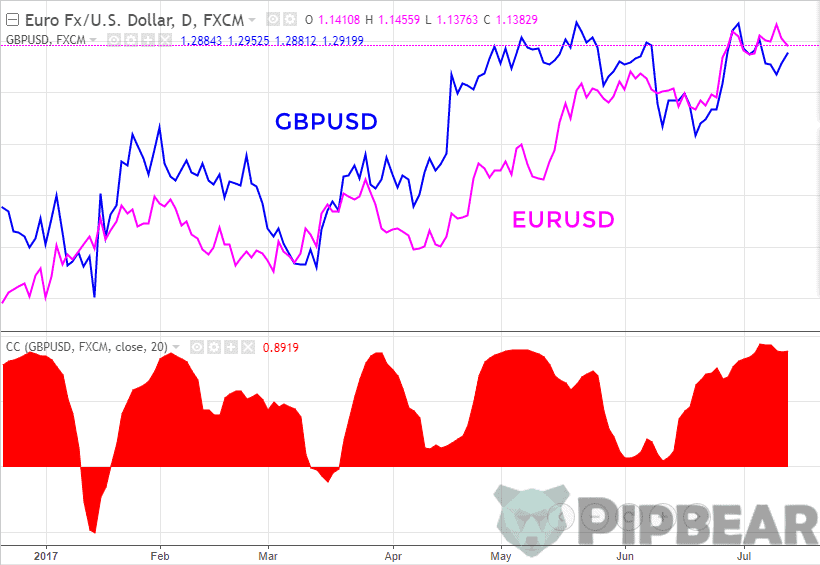If the correlation is negative, the tools move in opposite directions. Look at the EURUSD and USDCHF currency pairs. Both cases are examples of a strong dependence.You trade the spread pairs in case of pairs trading, that is, the difference between these two tools. Since we know that the tool moves in one direction, it means that they will most likely come back together after the next divergence.

The easiest way to illustrate the pairs trading strategy is based on the EURUSD and GBPUSD pairs. When the spread (difference) between the two tools is widening to a certain threshold, we should buy the lagging tool and sell the leading one. When the tools come together again, we must lock in profits.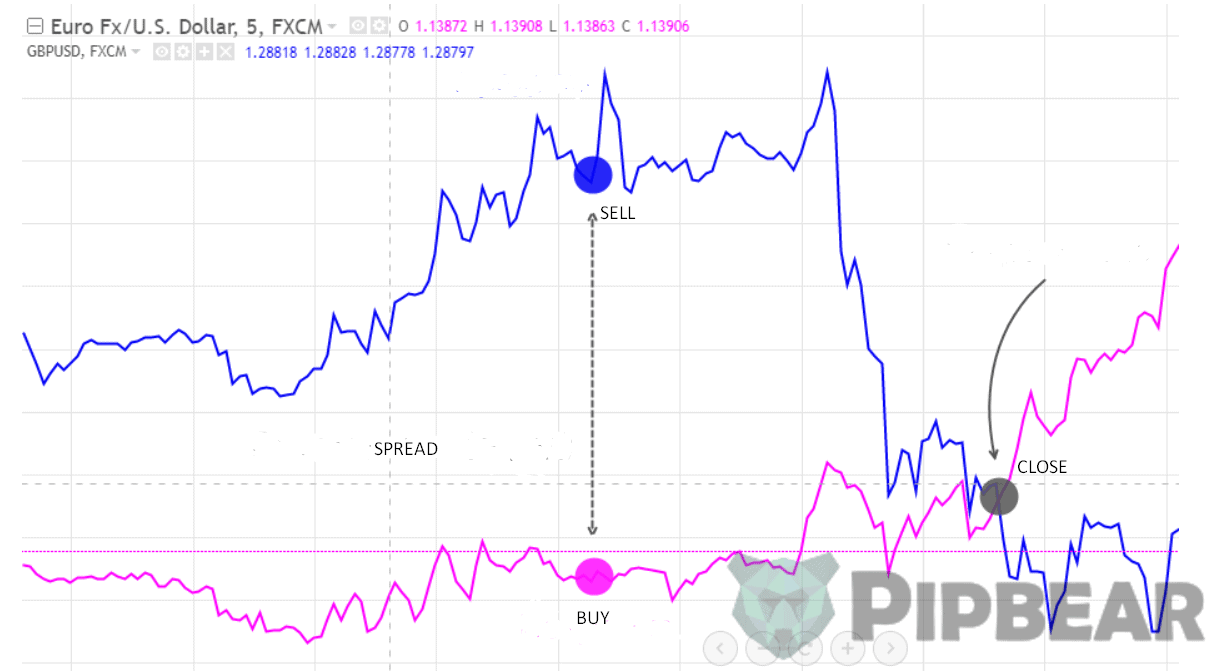The significance of the threshold divergence is determined by the statistical method and analysis of the previous divergence history. For example, it can be an average divergence over the last year.

After all, it doesn’t matter which direction a separate tool will go to. What matters is the tool to come together again, so their spread should be back to zero. At this point, we lock in profits equal to the divergence size.

This strategy will be profitable if there is a constant interrelation between the tools. The EURUSD and GBPUSD pairs have a fairly strong positive correlation. However, this dependence is not constant. Therefore, the pair can diverge for a very long distance and fail to converge back.

If the pair had a permanent strong correlation, the EURGBP would always be flat. However, dependence collapses from time to time and that is when the trends are formed.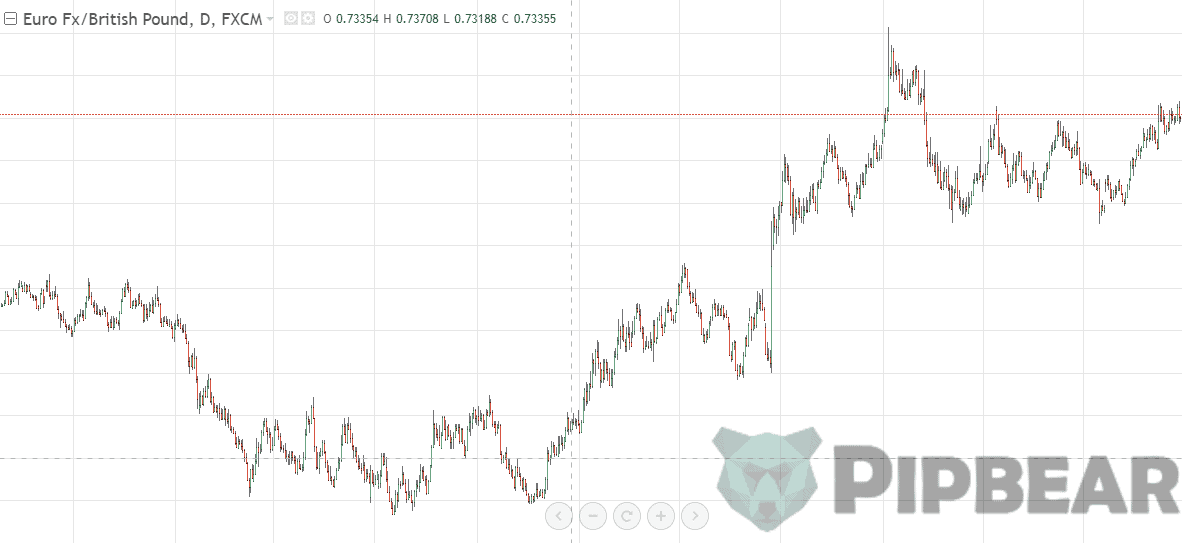Let’s go now to practice and create the spread of two tools. We shall use the TradingView service to conduct calculations of the spread between these tools.

There are different ways of calculating the spread, which brings slightly different results. You may choose the best one down to your preference. The simplest way is to calculate the spread by the difference. That is, the spread formula for EURUSD and GBPUSD will look like EURUSD – GBPUSD.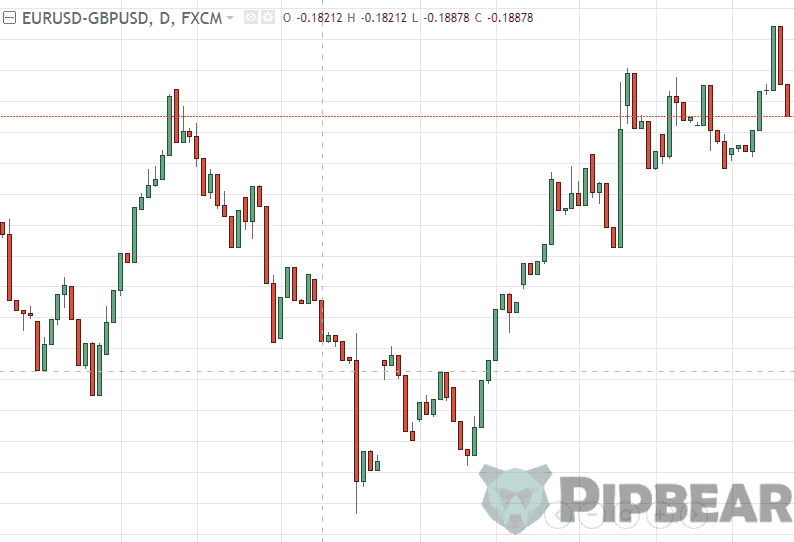You can also create a spread about, for example, EURUSD / GBPUSD. You should take into account that the final signals may vary depending on the chosen method but they won’t be fundamentally different.

After that, you need to decide at which moment you shall open the position. The thing is to enter the market when the spread is expanding fast, that is when the tools are temporarily losing the correlation between them. Strong spread expansion stands for the expansion exceeding the average one. Therefore, the difference between the spread chart and the moving average can be a good indicator for the entry.

Thus, we will get the spread oscillator. It is very simple to trade this spread. When the line goes into the overbought zone (the spread has gone too far from the average values), we should sell the spread. When it enters the oversold zone, we should turn over the position.

In this case, we need to buy EURUSD and sell GBPUSD to buy the spread. And if we want to sell the spread, we should sell the euro and buy pounds.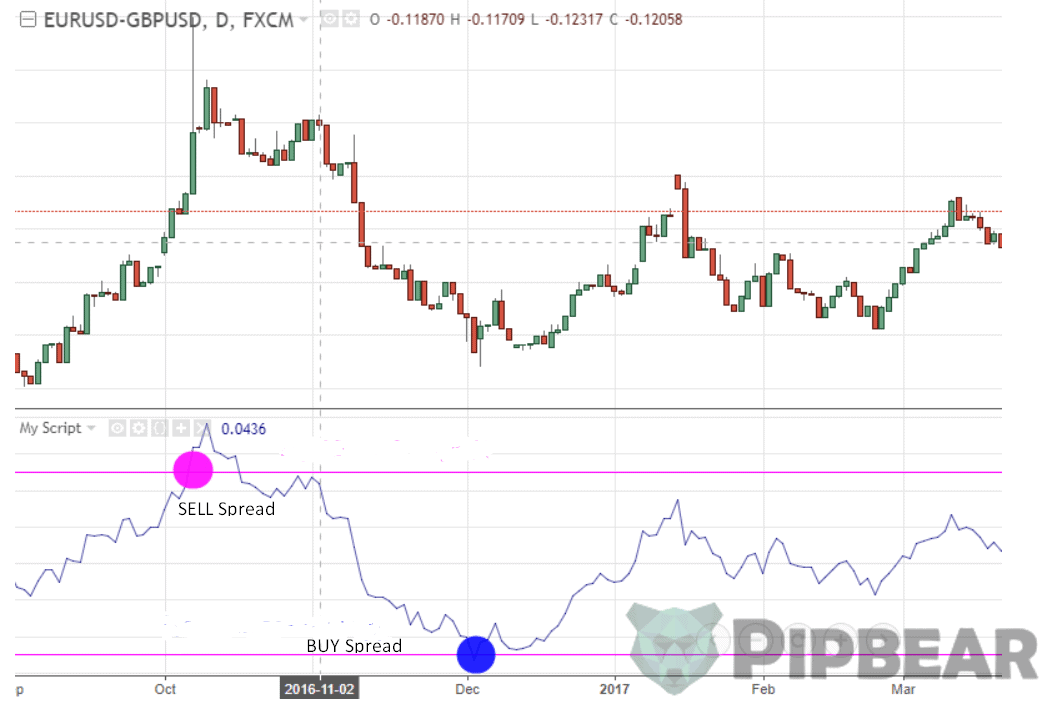Open the “Pine Editor” and add the line “plot (close-sma (close, 50))” to build a chart of this kind. That is, we should deduct the moving average with a period of 50 from the current closing price. Click “Add to Cart” to add the oscillator to the chart.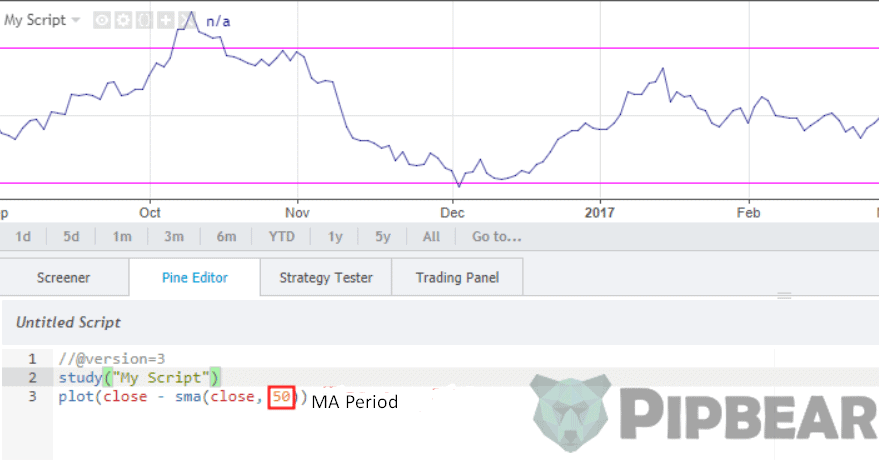Another method is to trade spread from the borders of the Bollinger Channel. All you need to do so is to add the Bollinger Bands indicator to the spread chart. In this case, the crossing of the channel borders will also indicate a significant deviation of the spread from the average one.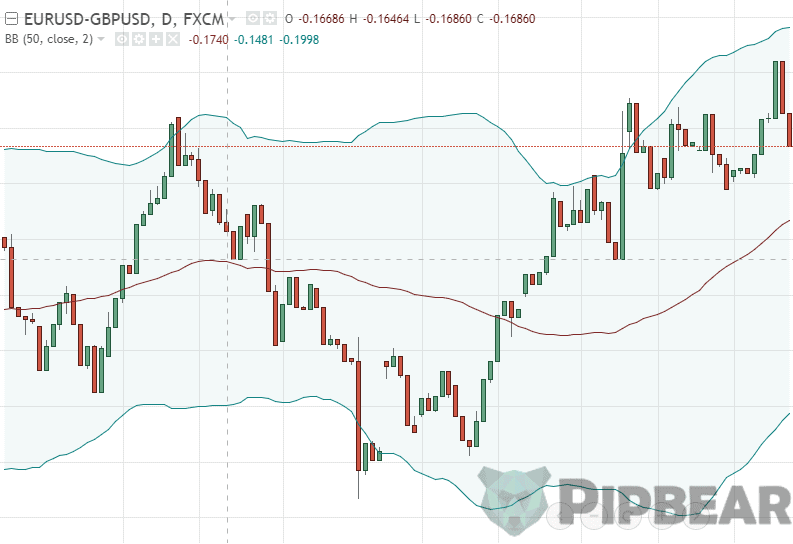## Calculating the order size

Another important thing is the correct calculation of the size of the order for the position of the pair. It is quite logical to assume that two orders should be of equal volume so it shall be enough to open two positions of the same number of the lots. However, it is not that simple. If we calculate the divergence between the tools in points, then we may assume that the points are equal for both of the tools.

We should take into account different cost of the point of two tools relative to the dollar to balance the positions. You can find the cost of a currency pair point by using point cost calculator.

Let’s say you want to open a pair position for EURUSD and USDJPY. The point cost for EURUSD is \$1. The point cost for USDJPY at the moment is equal to \$0.87788. So if we need to equalize the sizes of the positions, we should take a 1.14 times larger USDJPY position than the one for EURUSD (1 / 0.87788).

## Conclusion

The most important part of the pairs trading strategy is to pick the trading tools properly. You should understand that the strategy itself is not a Holy Grail but it may bring you a stable low-risk profit if the correlating assets are chosen correctly. If you want to study statistical arbitrage, you should start with pairs trading.